Processing ......FreeComputerBooks.com Links to Free Computer, Mathematics, Technical Books all over the World

Applied Combinatorics
🌠 Top Free Data Science Books - 100% Free or Open Source!
• Title: Applied Combinatorics
• Author(s) Mitchel T. Keller, William T. Trotter
• Publisher: CreateSpace (2017); eBook (Creative Commons Licensed, 2017)
• Paperback: 392 pages
• eBook: HTML and PDF (393 pages)
• Language: English
• ISBN-10: 1534878653
• ISBN-13: 978-1534878655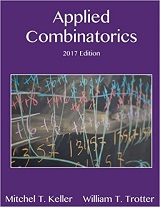Book Description

This is a text with more than enough material for a one-semester introduction to combinatorics. The original target audience was primarily computer science majors, but the topics included make it suitable for a variety of different students. Topics include:

• Basic enumeration: strings, sets, binomial coefficients
• Recursion and mathematical induction
• Graph theory
• Partially ordered sets
• Additional enumeration techniques: inclusion-exclusion, generating functions, recurrence relations, and Polya theory.
• Graph algorithms: minimum weight spanning trees, Dijkstra's algorithm, network flows

This text is open source and available under a Creative Commons license. To access the free HTML and PDF versions of the text, visit http://rellek.net/appcomb/.

• Mitchel T. Keller is an Assistant Professor of Mathematics at Washington and Lee University in Lexington, Virginia.
• William T. Trotter is a Professor of Mathematics at the Georgia Institute of Technology in Atlanta.
Reviews, Ratings, and Recommendations: Related Book Categories: Read and Download Links:Similar Books:
•Combinatorics Through Guided Discovery (Kenneth P. Bogart)

This book is an introduction to combinatorial mathematics, also known as combinatorics. The book focuses especially but not exclusively on the part of combinatorics that mathematicians refer to as 'counting'.

•Analytic Combinatorics (Philippe Flajolet, et al)

The definitive treatment of analytic combinatorics. This self-contained text covers the mathematics underlying the analysis of discrete structures, with thorough treatment of a large number of applications.

•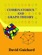An Introduction to Combinatorics and Graph Theory

This book walks the reader through the classic parts of Combinatorics and graph theory, while also discussing some recent progress in the area: on the one hand, providing material that will help students learn the basic techniques.

•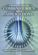Foundations of Combinatorics with Applications (Edward Bender)

This introduction to combinatorics, the foundation of the interaction between computer science and mathematics, is suitable for upper-level undergraduates and graduate students in engineering, science, and mathematics.

•Lists, Decisions and Graphs - With an Introduction to Probability

In this book, four basic areas of discrete mathematics are presented: Counting and Listing (Unit CL), Functions (Unit Fn), Decision Trees and Recursion (Unit DT), and Basic Concepts in Graph Theory (Unit GT).

•Magic Squares and Cubes (William Symes Andrews)

This book cover topics such as magic squares, magic cubes, the Franklin squares, magics and Pythagorean numbers, the theory of reversions, magic circles, spheres, and stars, and magic octahedroids, among other things.

•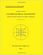Combinatorial Geometry with Application to Field Theory

Topics covered in this book include fundamental of combinatorics, algebraic combinatorics, topology with Smarandache geometry, combinatorial differential geometry, combinatorial Riemannian submanifolds, Lie multi-groups, etc.

•Notes on Convex sets, Polytopes, Polyhedra, Combinatorial Topology

This book may be viewed as a tutorial and a set of notes on convex sets, polytopes, polyhedra, combinatorial topology, Voronoi Diagrams and Delaunay Triangulations.

•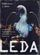LEDA: A Platform for Combinatorial and Geometric Computing

LEDA is a library of efficient data types and algorithms and a platform for combinatorial and geometric computing. This book, written by the main authors of LEDA, is the definitive account of how the system operates and how it can be used.

•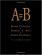A=B, by Marko Petkovsek, Herbert S. Wilf, et al

This book is of interest to mathematicians and computer scientists working in finite mathematics and combinatorics. It presents a breakthrough method for analyzing complex summations.

•Algebraic Combinatorics on Words (M. Lothaire)

This book is both a comprehensive introduction to the subject and a valuable reference source for researchers.

•Applied Combinatorics on Words (M. Lothaire)

The aim of this book is to present a unified treatment of some of the major fields of applications of combinatorics.

•Combinatorial Algorithms for Computers and Calculators (H. S. Wilf)

On one level, this is a collection of subroutines, in FORTRAN, for the solution of combinatorial problems.

•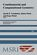Combinatorial and Computational Geometry (Jacob E. Goodman)

It includes surveys and research articles exploring geometric arrangements, polytopes, packing, covering, discrete convexity, geometric algorithms and their complexity, and the combinatorial complexity of geometric objects, particularly in low dimension.

•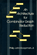Architecture for Combinator Graph Reduction (Philip J. Koopman)

The results of cache-simulation experiments with an abstract machine for reducing combinator graphs are presented.

•Knapsack Problems: Algorithms and Computer Implementations

The text fully develops an algorithmic approach to Knapsack Problems without losing mathematical rigor.

•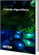Greedy Algorithms (Witold Bednorz)

This book covers fundamental, theoretical topics as well as advanced, practical applications of Greedy Algorithms.

Book Categories
 :All CategoriesTop Free BooksRecent BooksMiscellaneous BooksComputer EngineeringComputer LanguagesComputer ScienceData Science/DatabasesJava and Java EE (J2EE)Linux and UnixMathematicsMicrosoft and .NETMobile ComputingNetworking and CommunicationsSoftware EngineeringSpecial TopicsWeb Programming
Other Categories